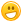y.radev 6 Точки

## 08. Recursive Fibonacci

Здравейте, някой може ли да ми открие грешката в моето решение.

Условие:

## 1.Recursive Fibonacci

Each member of the Fibonacci sequence is calculated from the sum of the two previous members. The first two elements are 1, 1. Therefore the sequence goes as 1, 1, 2, 3, 5, 8, 13, 21, 34…

The following sequence can be generated with an array, but that’s easy, so your task is to implement it recursively.

If the function getFibonacci(n) returns the nth Fibonacci number, we can express it using getFibonacci(n) = getFibonacci(n-1) + getFibonacci(n-2).

However, this will never end and in a few seconds a Stack Overflow Exception is thrown. In order for the recursion to stop it has to have a "bottom". The bottom of the recursion is getFibonacci(1), and should return 1. The same goes for getFibonacci(0).

### Input

• On the only line in the input the user should enter the wanted Fibonacci number N where 1 ≤ N ≤ 49

### Output

The output should be the nth Fibonacci number counting from

Линк към задача: Stacks and Queues - Exercises - SoftUni Judge

Мое решение: https://pastebin.com/q16Y2zku -- 88/100 гърми тест 1

Благодаря за отделеното време!

Тагове:
0
23/08/2021 16:30:04svephoto 1188 Точки

В if-проверката на ред 12 остави само (N < 2) и си готов със 100/100.0y.radev 6 Точки

Благодаря ти! :D

1
Можем ли да използваме бисквитки?
Ние използваме бисквитки и подобни технологии, за да предоставим нашите услуги. Можете да се съгласите с всички или част от тях.
Назад
Функционални
Използваме бисквитки и подобни технологии, за да предоставим нашите услуги. Използваме „сесийни“ бисквитки, за да Ви идентифицираме временно. Те се пазят само по време на активната употреба на услугите ни. След излизане от приложението, затваряне на браузъра или мобилното устройство, данните се трият. Използваме бисквитки, за да предоставим опцията „Запомни Ме“, която Ви позволява да използвате нашите услуги без да предоставяте потребителско име и парола. Допълнително е възможно да използваме бисквитки за да съхраняваме различни малки настройки, като избор на езика, позиции на менюта и персонализирано съдържание. Използваме бисквитки и за измерване на маркетинговите ни усилия.
Рекламни
Използваме бисквитки, за да измерваме маркетинг ефективността ни, броене на посещения, както и за проследяването дали дадено електронно писмо е било отворено.